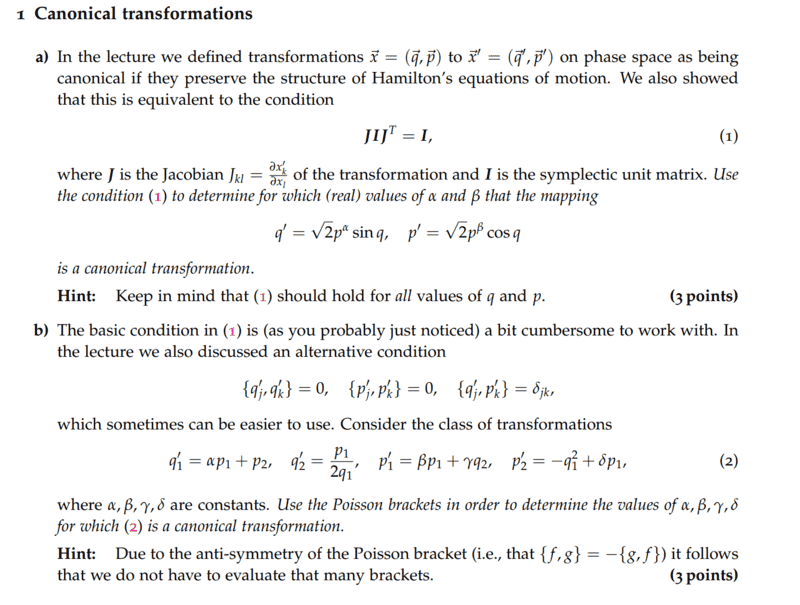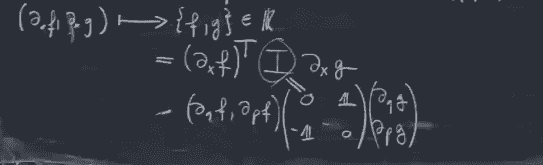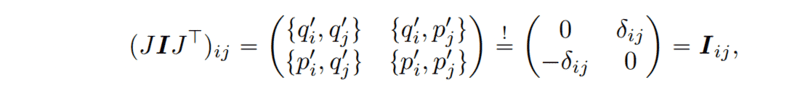# Canonical transformations

Lambda96
Homework Statement:
See Screenshot
Relevant Equations:
none
Hi,

unfortunately, I have problems with the following taskI first tried to calculate ##JIJ^T##.

$$\left( \begin{array}{rrr} \frac{\partial q'_i}{\partial q_j} & \frac{\partial q'_i}{\partial P_j} \\\frac{\partial P'_i}{\partial q_j} & \frac{\partial P'_i}{\partial P_j} \\ \end{array}\right)\left( \begin{array}{rrr} 0 & \textbf{1} \\\ -{\textbf{1}} & 0 \\ \end{array}\right)\left( \begin{array}{rrr} \frac{\partial q'_i}{\partial q_j} & \frac{\partial P'_i}{\partial P_j} \\\frac{\partial P'_i}{\partial q_j} & \frac{\partial q'_i}{\partial P_j} \\ \end{array}\right)=\left( \begin{array}{rrr} \frac{\partial q'_i}{\partial q_j} \textbf{1} \frac{\partial q'_i}{\partial P_j} -\frac{\partial q'_i}{\partial P_j} \textbf{1} \frac{\partial q'_i}{\partial q_j} & \frac{\partial q'_i}{\partial q_j} \textbf{1} \frac{\partial P_i}{\partial P_j} -\frac{\partial q'_i}{\partial P_j} \textbf{1} \frac{\partial P_i}{\partial q_j} \\ \frac{\partial P_i}{\partial q_j} \textbf{1} \frac{\partial q'_i}{\partial P_j} -\frac{\partial P_i}{\partial P_j} \textbf{1} \frac{\partial q'_i}{\partial q_j} & \frac{\partial P_i}{\partial q_j} \textbf{1} \frac{\partial P_i}{\partial P_j} -\frac{\partial P_i}{\partial P_j} \textbf{1} \frac{\partial P_i}{\partial q_j} \\ \end{array}\right)$$

##\textbf{1}## is supposed to be the unit matrix, but unfortunately I don't know how to write it with latex, which is why I have represented it in my calculation like this

Then I took the following relation from my professor, I hope you can read it well.and get the following
$$\left( \begin{array}{rrr} \Bigl\{ q'_i,q'_i \Bigr\} & \Bigl\{ q'_i,P_i \Bigr\} \\ \Bigl\{ P_i,q'_i \Bigr\} & \Bigl\{ P_i,P_i \Bigr\} \\ \end{array}\right)$$

Unfortunately, I am not getting anywhere now, because in order to show which values ##\alpha## and ##\beta## must assume in order for it to be a canonical transformation, I would have to get the symplectic unit matrix again, but with my calculation I would only get numbers as entries in the matrix and not unit matrices, as it should be.

My professor's script contains the following formulation•PhDeezNutz

Homework Helper
Gold Member
2022 Award
Hi @Lambda96. I’m no expert, but (since you haven’t any other replies and I may have seen where you are going wrong) here goes...

What you have written suggests that you are trying to apply a general format to construct equations for systems with 2 or more degrees of freedom. But the problem is simpler than that.

The given transformation equations (for ##p’## and ##q’##) show the system has only one degree of freedom (so the phase space is 2-dimensional).

That means ##\vec x## has only two components, ##x_1 = q## and ##x_2 = p##.

Similarly ##\vec x’## has only two components, ##x_1’= q’## and ##x_2’ = p’##.

The Jacobian tranformation matrix,##J##, is 2x2.

For example ##J_{11} = \frac {∂x_1’}{∂x_1} = \frac {∂q’}{∂q}##

Since ##q’ = \sqrt 2 p^α \sin q ## then ##J_{11} = \frac {∂q’}{∂q}= \sqrt 2 p^ α \cos q##

Similarly, you need to find ##J_{12}, J_{21}## and ##J_{22}##.

The corresponding symplectic identity matrix, ##I##, is also a 2x2 matrix (the elements are simple integers).

You are dealing only with 2x2 matrices (but still probably have some messy working ahead!). Multiply-out ##JIJ^T## and then find what values of ##\alpha## and ##\beta## are needed to make ##JIJ^T=I##.

•PhDeezNutz and Lambda96
Gold Member
2022 Award
The matrix notation is a bit clumsy when it comes to concrete calculations in my opinion. It's nice for formal calculations. It just says that the "canonical Poisson brackets" should hold for the new phase-space coordinates, i.e., you have to find values for ##\alpha## and ##\beta## such that
$$\{x',x' \}=\{p',p'\}=0, \quad \{x',p'\}=1.$$

•PhDeezNutz and Lambda96
Lambda96
Thanks for your help Steve4Physics, I had completely misunderstood the meaning of the matrix and its entries then, also thanks to vanhees71 for your help.

I have now done the calculation again:

$$\left( \begin{array}{rrr} \frac{\partial q'}{\partial q} & \frac{\partial q'}{\partial p} \\ \frac{\partial p'}{\partial q} & \frac{\partial p'}{\partial p} \\ \end{array}\right)\left( \begin{array}{rrr} 0 & 1 \\ -1 & 0 \\ \end{array}\right)\left( \begin{array}{rrr} \frac{\partial q'}{\partial q} & \frac{\partial p'}{\partial q} \\ \frac{\partial q'}{\partial p}& \frac{\partial p'}{\partial p} \\ \end{array}\right)$$

Inserting values then gives:

$$\left( \begin{array}{rrr} \frac{\partial q'}{\partial q} & \frac{\partial q'}{\partial p} \\ \frac{\partial p'}{\partial q} & \frac{\partial p'}{\partial p} \\ \end{array}\right)\left( \begin{array}{rrr} 0 & 1 \\ -1 & 0 \\ \end{array}\right)\left( \begin{array}{rrr} \frac{\partial q'}{\partial q} & \frac{\partial p'}{\partial q} \\ \frac{\partial q'}{\partial p}& \frac{\partial p'}{\partial p} \\ \end{array}\right)=\left( \begin{array}{rrr} \sqrt{2}p^{\alpha}\cos(q) & -\sqrt{2}p^{\beta}\sin(q) \\ \sqrt{2} \alpha p^{\alpha-1}\sin(q) & \sqrt{2} \beta p^{\beta-1}\cos(q) \ \end{array}\right) \left( \begin{array}{rrr} \sqrt{2} \alpha p^{\alpha-1} \sin(q) & -\sqrt{2}p^{\beta} \sin(q) \\ -\sqrt{2}p^{\alpha} \cos(q) & \sqrt{2} \beta p^{\beta-1} \cos(q) \ \end{array}\right)$$

$$=\left( \begin{array}{rrr} 2 \alpha p^{2\alpha-1} \cos(q) \sin(q) -2 \alpha p^{2\alpha -1} \cos(q) \sin(q) \ & 2 \beta p^{\alpha} \cos^2(1)p^{\beta -1} +2 \alpha p^{\beta} \sin^2(q)p^{\alpha -1} \\ 2 \alpha p^{\beta} \cos^2(1)p^{\alpha -1} -2 \beta p^{\alpha} \sin^2(q)p^{\beta -1} & -2 \beta p^{2\beta-1} \cos(q) \sin(q) +2 \beta p^{2\beta -1} \cos(q) \sin(q) \ \end{array}\right)$$

Now the following holds:

$$a_{11}: 2 \alpha p^{2\alpha-1} \cos(q) \sin(q) -2 \alpha p^{2\alpha -1} \cos(q) \sin(q) =0$$
$$a_{12}: 2 \beta p^{\alpha} \cos^2(1)p^{\beta -1} +2 \alpha p^{\beta} \sin^2(q)p^{\alpha -1}=1$$
$$a_{21}: 2 \alpha p^{\beta} \cos^2(1)p^{\alpha -1} -2 \beta p^{\alpha} \sin^2(q)p^{\beta -1} =-1$$
$$a_{22}: -2 \beta p^{2\beta-1} \cos(q) \sin(q) +2 \beta p^{2\beta -1} \cos(q) \sin(q)=0$$

So now I just have to solve the 4 equations for ##\alpha## and ##\beta##.

•Steve4Physics
Gold Member
2022 Award
Are all these equations independent from each other? Are all of them give any restrictions on ##\alpha## and ##\beta##? Finally note that the right-hand sides of the equations are independent of ##q## and ##p##. What does this imply for ##\alpha## and ##\beta##?

•Lambda96
Homework Helper
Gold Member
2022 Award
So now I just have to solve the 4 equations for ##\alpha## and ##\beta##.
Well, only 2 equations really. On inspection, the values of ##a_{11}## and ##a_{22}## are necessarily both zero.

I haven't checked you working but you appear to have some typo's: ##a_{12}## and ##a_{21}## contain ##cos^2(1)## which doesn't look right.

More generally, if you think it would be useful, the video below provides a wonderful (IMHO) treatment of material relevant to your Post #1 question.

However, the video is 1h 18m long (excluding the YouTube ad’s). If you watch it, be prepared to regularly hit the ‘Skip ad’ button! And don’t be put-off by the quite-basic first 5 minutes.

•Lambda96
Lambda96
Thanks Steve4Physics and vanhees71 for your help👍, thanks also for the videos 👍

@Steve4Physics I have now checked my calculation several times regarding ##a_{12}## and ##a_{21}## but I always get ##cos^2## and ##sin^2## or do you mean that the 1 in ##cos^2## and ##sin^2## is wrong? If so, that's right it should actually be ##cos^2(q)## and ##sin^2(q)##, I don't know how the 1 got in theirHomework Helper
Gold Member
2022 Award
If so, that's right it should actually be ##cos^2(q)## and ##sin^2(q)##, I don't know how the 1 got in theirYes. You are given ##q’ = \sqrt 2 p^α \sin (q) ## and ##p’ = \sqrt 2 p^ β \cos (q) ##.

When you differentiate, you can only get expressions containing ##\cos (q)## and ##\sin (q) ##. Terms containing ##\sin (1) ## and/or ##\cos (1) ## can't arise. Looks like some sort of transcription error that turned ‘##q##’ into ‘##1##’.

If that’s the only mistake, I’d guess that you should have
##a_{12}: 2 \beta p^{\alpha} \cos^2(q)p^{\beta -1} +2 \alpha p^{\beta} \sin^2(q)p^{\alpha -1}=1##
##a_{21}: 2 \alpha p^{\beta} \cos^2(q)p^{\alpha -1} -2 \beta p^{\alpha} \sin^2(q)p^{\beta -1} =-1##

These can be simplified/combined in different ways. But I'll admit I don't see how to find ##\alpha## and ##\beta## from them.

•Lambda96
Gold Member
2022 Award
Some hints: First of all there's only one non-trivial Poisson bracket here, which is ##a_{12}=-a_{21}## the other are ##0##, because ##\{Q,Q\}=\{P,P\}## for all phase-space functions ##Q(q,p)## and ##P(q,p)##.

Finally you need two equations to solve for ##\alpha## and ##\beta##. After simplifying your equations for ##a_{12}## a bit, you'll see that one equation follows from the demand that the right-hand side must not depend on ##p##. After solving for this, ##a_{12}=1## gives the 2nd equation.

•Lambda96, Steve4Physics and vela
Staff Emeritus
Homework Helper
But I'll admit I don't see how to find ##\alpha## and ##\beta## from them.
After eliminating the dependence on ##p##, rather than trying to solve for ##\alpha## or ##\beta## individually, think about what pair of values will work to get rid of the dependence on ##q##.

•Lambda96, vanhees71 and Steve4Physics
Homework Helper
Gold Member
$$\left( \begin{array}{rrr} \frac{\partial q'}{\partial q} & \frac{\partial q'}{\partial p} \\ \frac{\partial p'}{\partial q} & \frac{\partial p'}{\partial p} \\ \end{array}\right)\left( \begin{array}{rrr} 0 & 1 \\ -1 & 0 \\ \end{array}\right)\left( \begin{array}{rrr} \frac{\partial q'}{\partial q} & \frac{\partial p'}{\partial q} \\ \frac{\partial q'}{\partial p}& \frac{\partial p'}{\partial p} \\ \end{array}\right)$$
Instead of substituting the explicit expressions for ##q'## and ##p'## in terms of ##q## and ##p## at this point, I think it's much nicer to carry out the matrix multiplication above and show that it reduces to what your professor indicated:
$$\left( \begin{array}{rrr} \{q', q'\} &\{q', p'\} \\ \{p', q'\} & \{p', p'\} \\ \end{array}\right)$$ It should be easy to see that two of the entries here are zero and the other two differ only in sign. Since the matrix is required to equal the matrix
$$\left( \begin{array}{rrr} 0 & 1 \\ -1 & 0 \\ \end{array}\right)$$ you end up with only one independent equation to be satisfied. This equation is your ##a_{12}## equation given below

$$a_{12}: 2 \beta p^{\alpha} \cos^2(1)p^{\beta -1} +2 \alpha p^{\beta} \sin^2(q)p^{\alpha -1}=1$$
Your ##a_{21}## equation has a sign error. The ##a_{21}## equation should be equivalent to the ##a_{12}## equation.

So, you just need to find values of ##\alpha## and ##\beta## such that the ##a_{12}## equation is satisfied.

•Lambda96 and vanhees71
Homework Helper
Gold Member
2022 Award
After eliminating the dependence on ##p##, rather than trying to solve for ##\alpha## or ##\beta## individually, think about what pair of values will work to get rid of the dependence on ##q##.
Thanks. I realised how to do it after reading @vanhees71's Post #9.

•vanhees71
Lambda96
Thank you Steve4Physics, vanhees71, vela and TSny for your help👍 👍 👍👍

I have now started to rewrite ##a_{12}## as follows:

$$2p^{\alpha} \cos^2(q) \beta p^{\beta -1}+2 \alpha p^{\alpha -1} \sin^2(q) p^{\beta}$$

$$2 \cos^2(q) \beta p^{\beta +\alpha -1}+ 2 \alpha p^{\alpha +\beta -1} \sin^2(q)$$

After all, I want p to vanish or rather become 1, which is the case when ##\alpha + \beta -1=0## simultaneously, I also want the 2 to vanish and become a 1. I would achieve all this if ##\alpha= \frac{1}{2}## and ##\beta=\frac{1}{2}##.

Regarding task b, I still have a question, if I have now understood correctly, the system has two degrees of freedom, must the Poisson bracket then be calculated as follows?

$$\Bigl\{ q'_j, p'_k \Bigr\}=\sum\limits_{i=1}^{2}\frac{\partial q'_j}{\partial q_i}\frac{\partial p'_k}{\partial p_i}- \frac{\partial q'_j}{\partial p_i}\frac{\partial p'_k}{\partial q_i}=\frac{\partial q'_j}{\partial q_1}\frac{\partial p'_k}{\partial p_1}- \frac{\partial q'_j}{\partial p_1}\frac{\partial p'_k}{\partial q_1}+\frac{\partial q'_j}{\partial q_2}\frac{\partial p'_k}{\partial p_2}-\frac{\partial q'_j}{\partial p_2}\frac{\partial p'_k}{\partial q_2}$$

I have tried to determine ##\alpha, \beta, \delta, \gamma## by calculating the following

$$\Bigl\{ q'_1,p'_1 \Bigr\}=1$$
$$\Bigl\{ q'_2,p'_2 \Bigr\}=1$$
$$\Bigl\{ q'_1,q'_2 \Bigr\}=0$$
$$\Bigl\{ p'_1,p'_2 \Bigr\}=0$$

With this I found out that ##\gamma=1##, ##\alpha=0##, ##\beta=0## and ##\delta=0## but since I got so many zeros I don't know if I defined the Poisson brackets correctly?

Homework Helper
Gold Member
2022 Award
Regarding task b, I still have a question, if I have now understood correctly, the system has two degrees of freedom, must the Poisson bracket then be calculated as follows?

$$\Bigl\{ q'_j, p'_k \Bigr\}=\sum\limits_{i=1}^{2}\frac{\partial q'_j}{\partial q_i}\frac{\partial p'_k}{\partial p_i}- \frac{\partial q'_j}{\partial p_i}\frac{\partial p'_k}{\partial q_i}=\frac{\partial q'_j}{\partial q_1}\frac{\partial p'_k}{\partial p_1}- \frac{\partial q'_j}{\partial p_1}\frac{\partial p'_k}{\partial q_1}+\frac{\partial q'_j}{\partial q_2}\frac{\partial p'_k}{\partial p_2}-\frac{\partial q'_j}{\partial p_2}\frac{\partial p'_k}{\partial q_2}$$
That looks ok to me.

Sorry, but I don't have the time to check your values for ##\alpha, \beta, \gamma## and ##\delta##.

•Lambda96
Homework Helper
Gold Member
Thank you Steve4Physics, vanhees71, vela and TSny for your help👍 👍 👍👍

I have now started to rewrite ##a_{12}## as follows:

$$2p^{\alpha} \cos^2(q) \beta p^{\beta -1}+2 \alpha p^{\alpha -1} \sin^2(q) p^{\beta}$$

$$2 \cos^2(q) \beta p^{\beta +\alpha -1}+ 2 \alpha p^{\alpha +\beta -1} \sin^2(q)$$

After all, I want p to vanish or rather become 1, which is the case when ##\alpha + \beta -1=0## simultaneously, I also want the 2 to vanish and become a 1. I would achieve all this if ##\alpha= \frac{1}{2}## and ##\beta=\frac{1}{2}##.
Looks good to me.

Regarding task b, I still have a question, if I have now understood correctly, the system has two degrees of freedom, must the Poisson bracket then be calculated as follows?

$$\Bigl\{ q'_j, p'_k \Bigr\}=\sum\limits_{i=1}^{2}\frac{\partial q'_j}{\partial q_i}\frac{\partial p'_k}{\partial p_i}- \frac{\partial q'_j}{\partial p_i}\frac{\partial p'_k}{\partial q_i}=\frac{\partial q'_j}{\partial q_1}\frac{\partial p'_k}{\partial p_1}- \frac{\partial q'_j}{\partial p_1}\frac{\partial p'_k}{\partial q_1}+\frac{\partial q'_j}{\partial q_2}\frac{\partial p'_k}{\partial p_2}-\frac{\partial q'_j}{\partial p_2}\frac{\partial p'_k}{\partial q_2}$$

I have tried to determine ##\alpha, \beta, \delta, \gamma## by calculating the following

$$\Bigl\{ q'_1,p'_1 \Bigr\}=1$$
$$\Bigl\{ q'_2,p'_2 \Bigr\}=1$$
$$\Bigl\{ q'_1,q'_2 \Bigr\}=0$$
$$\Bigl\{ p'_1,p'_2 \Bigr\}=0$$
There are some more brackets that you need to evaluate, such as ##\Bigl\{ q'_1,p'_2 \Bigr\}##.

With this I found out that ##\gamma=1##, ##\alpha=0##, ##\beta=0## and ##\delta=0## but since I got so many zeros I don't know if I defined the Poisson brackets correctly?

Last edited:
•Lambda96
Lambda96
That looks ok to me.

Sorry, but I don't have the time to check your values for ##\alpha, \beta, \gamma## and ##\delta##.
No problem, you have already helped me a lot with task 1 👍

Thank you TSny for your help 👍👍👍

I have checked my calculation for ##\gamma## again and unfortunately overlooked the minus sign, so the following applies ##\gamma=-1##

unfortunately I did not have so much time yesterday to add my complete calculation, my calculations are as follows

$$\Bigl\{ q'_1,p'_1 \Bigr\}=0 * \beta - \alpha * 0 + 0*0-1* \gamma=-\gamma \rightarrow \gamma=-1$$
$$\Bigl\{ q'_2,p'_2 \Bigr\}=-\frac{p_1}{2q_1^2} \delta + 1 + 0*0-0*0=-\frac{p_1}{2q_1^2} \delta + 1 \rightarrow \delta=0$$
$$\Bigl\{ q'_1,q'_2 \Bigr\}=-0 * \frac{p_1}{2q_1^2} + \alpha \frac{p_1}{2q_1^2} + 0*0-1*0=\alpha \frac{p_1}{2q_1^2} \rightarrow \alpha=0$$
$$\Bigl\{ p'_1,p'_2 \Bigr\}=0 * \delta + 2\beta q_1 + \gamma*0-0*0=2\beta q_1 \rightarrow \beta=0$$
$$\Bigl\{ q'_1,p'_2 \Bigr\}=0 * \delta +2 \alpha q_1 + 0*0-1*0=2 \alpha q_1 \rightarrow \alpha=0$$

Homework Helper
Gold Member
No problem, you have already helped me a lot with task 1 👍

Thank you TSny for your help 👍👍👍

I have checked my calculation for ##\gamma## again and unfortunately overlooked the minus sign, so the following applies ##\gamma=-1##

unfortunately I did not have so much time yesterday to add my complete calculation, my calculations are as follows

$$\Bigl\{ q'_1,p'_1 \Bigr\}=0 * \beta - \alpha * 0 + 0*0-1* \gamma=-\gamma \rightarrow \gamma=-1$$
$$\Bigl\{ q'_2,p'_2 \Bigr\}=-\frac{p_1}{2q_1^2} \delta + 1 + 0*0-0*0=-\frac{p_1}{2q_1^2} \delta + 1 \rightarrow \delta=0$$
$$\Bigl\{ q'_1,q'_2 \Bigr\}=-0 * \frac{p_1}{2q_1^2} + \alpha \frac{p_1}{2q_1^2} + 0*0-1*0=\alpha \frac{p_1}{2q_1^2} \rightarrow \alpha=0$$
$$\Bigl\{ p'_1,p'_2 \Bigr\}=0 * \delta + 2\beta q_1 + \gamma*0-0*0=2\beta q_1 \rightarrow \beta=0$$
$$\Bigl\{ q'_1,p'_2 \Bigr\}=0 * \delta +2 \alpha q_1 + 0*0-1*0=2 \alpha q_1 \rightarrow \alpha=0$$
That all looks good.

•Lambda96
Lambda96
Thank you TSny, for your help and for looking over my calculations 👍👍👍

For ##\Bigl\{ q_2' , p_1' \Bigr\}## I have obtained the following:

$$\Bigl\{ q_2' , p_1' \Bigr\}=-\frac{p_1 \beta}{2q_1^2}-\frac{1}{2q_1}*0+0*0-0* \gamma=-\frac{p_1 \beta}{2q_1^2}=0 \rightarrow \beta=0$$

•TSny
Homework Helper
Gold Member
Thank you TSny, for your help and for looking over my calculations 👍👍👍

For ##\Bigl\{ q_2' , p_1' \Bigr\}## I have obtained the following:

$$\Bigl\{ q_2' , p_1' \Bigr\}=-\frac{p_1 \beta}{2q_1^2}-\frac{1}{2q_1}*0+0*0-0* \gamma=-\frac{p_1 \beta}{2q_1^2}=0 \rightarrow \beta=0$$
Yes. Nice work.

•Lambda96
Lambda96
Thanks for your help TSny and for looking over my calculation 👍👍👍

•TSny